# R语言入门系列之一

2019/02/17 13:19

1.对象与函数

Rhttps://cran.r-project.org/）是一个很好的数据分析以及可视化工具。R对象（object）是指可以赋值给变量（variable）的任何事物，在R语言中使用对象来存储数据也即储存变量，对象类型有标量、向量、矩阵、数组、数据框、列表。R语言通过函数（function）来提取对象属性、变量运算，函数可以来自R平台，也可以来自各种软件包（package）、自定义函数

R语言不用事先声明对象或变量，对象在赋值时同步创建。对象或变量名以字母开头，可由字母、数字、“.”“_”组成。对象赋值一般使用赋值符号“<-”，而在很多情况下也可以用传值符号“=”代替，也即“=”具有二义性，区别在于在函数内部时“=”只具有参数传递作用，举例如下：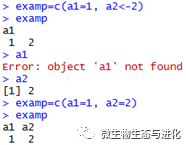在函数的括号内部使用“=”则将一个值传递给函数的内置参数（这个参数必须是函数具有的），而使用“<-”则将一个值赋值给变量，这时候a2作为一个对象而不是参数存在。可以使用objects()函数来查看当前对象目录，使用rm(objectname)来移除遗留的对象。

推荐在安装R后安装RStudio（https://www.rstudio.com/），在RStudio中编辑、运行R脚本。

1.1标量与向量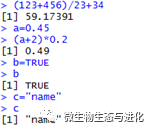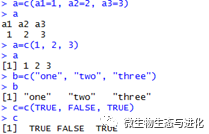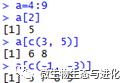R语言中判断符号有大于“>”、大于等于“>=”、小于“<”、小于等于“<=”、完全等于“==”、不等于“!=”、存在于“%in%”，如下所示：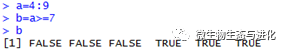vector(mode="logical", length=0)

numeric(length=0)

character(length=0)

as.vector(x, mode="any")

is.vector(x,mode="any")

rep(x, times=0) #产生重复，x可以是任意标量、向量，times为重复次数

seq(1.5, 6, by=0.5) #产生序列数，前面两个数为起始范围，by为步长

sample(x, size=3, replace=FALSE) #x（任意向量）中随机抽样，size抽样次数，replace是否放回抽样

pretty(c(a, b), n) #将区间(a, b)插入n个等间距的间隔点，从而将区间分成n+1个相等区域，在画图中常用

R可以非常灵活的处理数值与文本数据，并且有很好的面向对象的编程方式，对于标量与向量，常用内置基本函数如下所示（其中绿色部分为数值处理；蓝色部分为字符串处理，R支持正则表达式；红色部分为对象属性及操作）：

 函数 含义 round() round(x,  2)将数值对象x四舍五入法保留小数点后2位 trunc() 四舍五入去整，floor()向下取整，ceiling()向上取整 signif() 取有效数字 sqrt() 返回标量或向量元素的平方根 log() log(x,  y)返回以y为底x的对数，y默认值自然常数e exp() 返回自然常数e的指数 sin() 返回正弦值，其余还有cos()、tan()等详细查看?sin min() 返回最小值，此外which.min()返回最小值id max() 返回最大值，此外which.max()返回最小值id abs() 返回数值对象绝对值 sum() 返回对象元素的和 prod() 返回对象元素的乘积 mean() 返回对象元素的均值 var() 返回对象元素的方差 sd() 返回对象元素的标准差 median() 返回对象元素的中位数 nchar() 返回标量或向量元素的字符长度（包含空格） paste() paste(a,b,c,sep="  ")将a、b、c粘贴为一个字符串，空格分割 tolower() 转换为小写，toupper()转换为大写 substring() substring(a,1,3)返回字符对象a中第1到第3个字符 strsplit() strsplit(x,  split=" ", fixed=FALSE, perl=FALSE)根据split将字符串对象x分割，默认split为正则表达式，  fixed=TRUE则做精确匹配，当perl=TRUE时，使用perl的正则表达式规则，当分隔符为?,  +, {, |, (, )时，要使用'\\'来消除特殊含义 grep() grep("x",  a)，返回a中包含有字符“x”的元素id，可以使用正则表达式匹配，与strsplit()类似。此外还有grepl()返回匹配逻辑值、sub()替换、gsub()全局替换等 length() 返回对象的长度也即元素个数 mode() 查看对象数据类型（也即数值型、字符型等） names() 返回向量元素名字 order() 对向量元素排序，decreasing=TRUE则为降序，na.last=TRUE将缺失值排在最后，返回值为元素排名 sort() 对对象元素排序（不限于向量），返回排序后的对象 union() union(a,  b)求两个向量并集 intersect() 求两个向量的交集 setdiff() setdiff(a,  b)求在a中而不在b中的部分 setequal() setequal(a,  b)检验ab是否完全相同，此外is.element(12,  a)检验元素12是否属于a，all(c%in%a)检验集合a是否包含c

R语言有一些内置常量，方便使用的时候调取：

LETTERS              #26个大写字母

letters                 #26个小写字母

month.abb          #12个月份的三字母缩写

month.name       #12个月份的全称

pi                        #3.14...

colors()               #语言颜色表

1.2矩阵与数组

matrix(vector, nrow=m, ncol=n) #使用向量生成mn列的矩阵

matrix(NA, nrow=m, ncol=n) #生成一个mn列的空矩阵

as.matrix(x) #将对象转换为矩阵

is.matrix(x) #判断对象是否为矩阵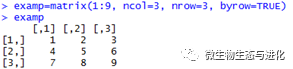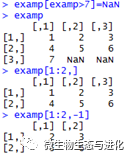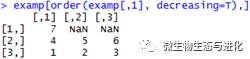ncol() 返回矩阵、数组、数据框的列数目 nrow() 返回行数目 colnames() 返回列名字 rownames() 返回行名字 t() 矩阵转置

array(vector, dimensions=c(3,3,3), dimnames=list(dim1,dim2,dim3)) #使用向量生成3*3*3的数组，dimnames为行名字、列名字、维度名字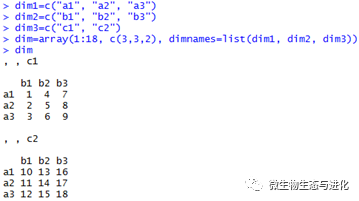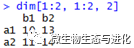1.3数据框与因子

data.frame(matrix, row.names=NULL, check.names=FALSE)

data.frame(col1, col2, col3...)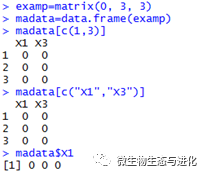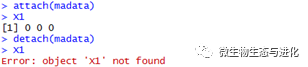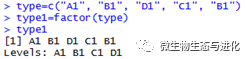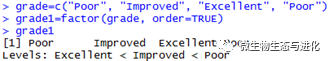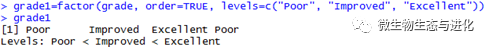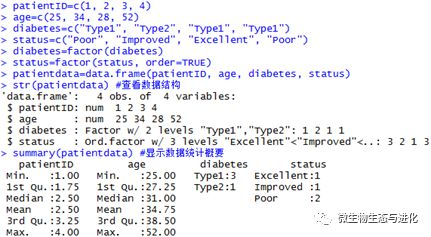1.4列表

list(object1, object2, ...) #默认名字为编号

list(name1=object1, name2=object2, ...) #为每个对象命名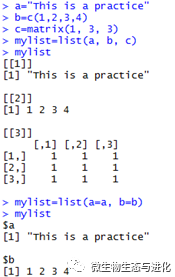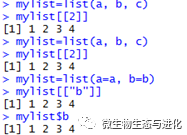1.5软件包

R语言提供了大量的功能，而且大部分功能是通过可选模块进行下载安装，这些模块被称为包（package）。这些包即有用来分析作图的函数包，也有用来作为例子的数据包。包即可以在线安装，也可以下载后本地安装。对于大部分包，均可以通过函数install.packages()来进行安装，如下所示：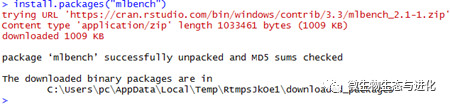source("https://bioconductor.org/biocLite.R")

biocLite("packagename")

devtools::install_github("class/packagename")

.packages(all.available=T)

installed.packages()[,c('Package','Version','LibPath')]

available.packages()

ap=available.packages()

grep('packagename', rownames(ap))

(.packages())

detach("package:packagename")

library(installr)

updateR()

1.6输入与输出

R可以通过键盘输入数据，也可以导入其他数据框软件生成的数据，常用的一般为文本文件、Excel文件、Web文件等。

mydata=data.frame(a1=numeric(0), a2=numeric(0), a3=character(0))

mydata=edit(mydata)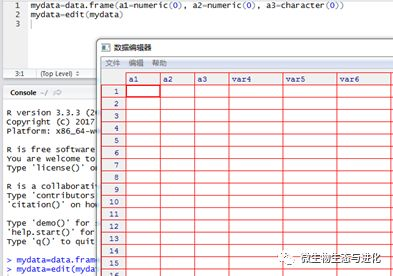row.names, col.names,na.strings = "NA",

colClasses =NA, check.names = TRUE

stringsAsFactors = default.stringsAsFactors())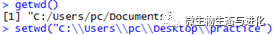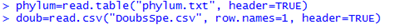write.table(object, file="filename", quote=F,row.names=F, col.names=T, sep='\t')

pdf("practice.pdf", width=9, height=9)

x=1:10

y=1:10

plot(x, y, type="p")

dev.off()

0
0 收藏

### 作者的其它热门文章0 评论
0 收藏
0